xLebesgue measureEncyclopedia
In measure theory
Measure (mathematics)
In mathematical analysis, a measure on a set is a systematic way to assign to each suitable subset a number, intuitively interpreted as the size of the subset. In this sense, a measure is a generalization of the concepts of length, area, and volume...

, the Lebesgue measure, named after French mathematician Henri Lebesgue
Henri Lebesgue
Henri Léon Lebesgue was a French mathematician most famous for his theory of integration, which was a generalization of the seventeenth century concept of integration—summing the area between an axis and the curve of a function defined for that axis...

, is the standard way of assigning a measure
Measure (mathematics)
In mathematical analysis, a measure on a set is a systematic way to assign to each suitable subset a number, intuitively interpreted as the size of the subset. In this sense, a measure is a generalization of the concepts of length, area, and volume...

to subset
Subset
In mathematics, especially in set theory, a set A is a subset of a set B if A is "contained" inside B. A and B may coincide. The relationship of one set being a subset of another is called inclusion or sometimes containment...

s of n-dimensional Euclidean space
Euclidean space
In mathematics, Euclidean space is the Euclidean plane and three-dimensional space of Euclidean geometry, as well as the generalizations of these notions to higher dimensions...

. For n = 1, 2, or 3, it coincides with the standard measure of length
Length
In geometric measurements, length most commonly refers to the longest dimension of an object.In certain contexts, the term "length" is reserved for a certain dimension of an object along which the length is measured. For example it is possible to cut a length of a wire which is shorter than wire...

, area
Area
Area is a quantity that expresses the extent of a two-dimensional surface or shape in the plane. Area can be understood as the amount of material with a given thickness that would be necessary to fashion a model of the shape, or the amount of paint necessary to cover the surface with a single coat...

, or volume
Volume
Volume is the quantity of three-dimensional space enclosed by some closed boundary, for example, the space that a substance or shape occupies or contains....

. In general, it is also called n-dimensional volume, n-volume, or simply volume. It is used throughout real analysis
Real analysis
Real analysis, is a branch of mathematical analysis dealing with the set of real numbers and functions of a real variable. In particular, it deals with the analytic properties of real functions and sequences, including convergence and limits of sequences of real numbers, the calculus of the real...

, in particular to define Lebesgue integration
Lebesgue integration
In mathematics, Lebesgue integration, named after French mathematician Henri Lebesgue , refers to both the general theory of integration of a function with respect to a general measure, and to the specific case of integration of a function defined on a subset of the real line or a higher...

. Sets that can be assigned a Lebesgue measure are called Lebesgue measurable; the measure of the Lebesgue measurable set A is denoted by λ(A).

Henri Lebesgue described this measure in the year 1901, followed the next year by his description of the Lebesgue integral. Both were published as part of his dissertation in 1902.

The Lebesgue measure is often denoted dx, but this should not be confused with the distinct notion of a volume form
Volume form
In mathematics, a volume form on a differentiable manifold is a nowhere-vanishing differential form of top degree. Thus on a manifold M of dimension n, a volume form is an n-form, a section of the line bundle Ωn = Λn, that is nowhere equal to zero. A manifold has a volume...

.

## Examples

• Any closed interval [a, b] of real number
Real number
In mathematics, a real number is a value that represents a quantity along a continuum, such as -5 , 4/3 , 8.6 , √2 and π...

s is Lebesgue measurable, and its Lebesgue measure is the length ba. The open interval (a, b) has the same measure, since the difference between the two sets consists only of the end points a and b and has measure zero.
• Any Cartesian product
Cartesian product
In mathematics, a Cartesian product is a construction to build a new set out of a number of given sets. Each member of the Cartesian product corresponds to the selection of one element each in every one of those sets...

of intervals [a, b] and [c, d] is Lebesgue measurable, and its Lebesgue measure is (ba)(dc), the area of the corresponding rectangle
Rectangle
In Euclidean plane geometry, a rectangle is any quadrilateral with four right angles. The term "oblong" is occasionally used to refer to a non-square rectangle...

.
• The Lebesgue measure of the set of rational numbers in an interval of the line is 0, although the set is dense
Dense set
In topology and related areas of mathematics, a subset A of a topological space X is called dense if any point x in X belongs to A or is a limit point of A...

in the interval.
• The Cantor set
Cantor set
In mathematics, the Cantor set is a set of points lying on a single line segment that has a number of remarkable and deep properties. It was discovered in 1875 by Henry John Stephen Smith and introduced by German mathematician Georg Cantor in 1883....

is an example of an uncountable set
Uncountable set
In mathematics, an uncountable set is an infinite set that contains too many elements to be countable. The uncountability of a set is closely related to its cardinal number: a set is uncountable if its cardinal number is larger than that of the set of all natural numbers.-Characterizations:There...

that has Lebesgue measure zero.
• Vitali set
Vitali set
In mathematics, a Vitali set is an elementary example of a set of real numbers that is not Lebesgue measurable, found by . The Vitali theorem is the existence theorem that there are such sets. There are uncountably many Vitali sets, and their existence is proven on the assumption of the axiom of...

s are examples of sets that are not measurable
Non-measurable set
In mathematics, a non-measurable set is a set whose structure is so complicated that it cannot be assigned any meaningful measure. Such sets are constructed to shed light on the notions of length, area and volume in formal set theory....

with respect to the Lebesgue measure. Their existence relies on the axiom of choice.

## Properties

The Lebesgue measure on Rn has the following properties:
1. If A is a cartesian product
Cartesian product
In mathematics, a Cartesian product is a construction to build a new set out of a number of given sets. Each member of the Cartesian product corresponds to the selection of one element each in every one of those sets...

of intervals
Interval (mathematics)
In mathematics, a interval is a set of real numbers with the property that any number that lies between two numbers in the set is also included in the set. For example, the set of all numbers satisfying is an interval which contains and , as well as all numbers between them...

I1 × I2 × ... × In, then A is Lebesgue measurable and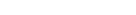Here, |I| denotes the length of the interval I.
2. If A is a disjoint union
Disjoint union
In mathematics, the term disjoint union may refer to one of two different concepts:* In set theory, a disjoint union is a modified union operation that indexes the elements according to which set they originated in; disjoint sets have no element in common.* In probability theory , a disjoint union...

of countably many disjoint Lebesgue measurable sets, then A is itself Lebesgue measurable and λ(A) is equal to the sum (or infinite series) of the measures of the involved measurable sets.
3. If A is Lebesgue measurable, then so is its complement
Complement (set theory)
In set theory, a complement of a set A refers to things not in , A. The relative complement of A with respect to a set B, is the set of elements in B but not in A...

.
4. λ(A) ≥ 0 for every Lebesgue measurable set A.
5. If A and B are Lebesgue measurable and A is a subset of B, then λ(A) ≤ λ(B). (A consequence of 2, 3 and 4.)
6. Countable unions
Union (set theory)
In set theory, the union of a collection of sets is the set of all distinct elements in the collection. The union of a collection of sets S_1, S_2, S_3, \dots , S_n\,\! gives a set S_1 \cup S_2 \cup S_3 \cup \dots \cup S_n.- Definition :...

and intersections
Intersection (set theory)
In mathematics, the intersection of two sets A and B is the set that contains all elements of A that also belong to B , but no other elements....

of Lebesgue measurable sets are Lebesgue measurable. (Not a consequence of 2 and 3, because a family of sets that is closed under complements and disjoint countable unions need not be closed under countable unions: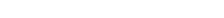.)
7. If A is an open
Open set
The concept of an open set is fundamental to many areas of mathematics, especially point-set topology and metric topology. Intuitively speaking, a set U is open if any point x in U can be "moved" a small amount in any direction and still be in the set U...

or closed
Closed set
In geometry, topology, and related branches of mathematics, a closed set is a set whose complement is an open set. In a topological space, a closed set can be defined as a set which contains all its limit points...

subset of Rn (or even Borel set
Borel set
In mathematics, a Borel set is any set in a topological space that can be formed from open sets through the operations of countable union, countable intersection, and relative complement...

, see metric space
Metric space
In mathematics, a metric space is a set where a notion of distance between elements of the set is defined.The metric space which most closely corresponds to our intuitive understanding of space is the 3-dimensional Euclidean space...

), then A is Lebesgue measurable.
8. If A is a Lebesgue measurable set, then it is "approximately open" and "approximately closed" in the sense of Lebesgue measure (see the regularity theorem for Lebesgue measure
Regularity theorem for Lebesgue measure
In mathematics, the regularity theorem for Lebesgue measure is a result in measure theory that states that Lebesgue measure on the real line is a regular measure...

).
9. Lebesgue measure is both locally finite
Locally finite measure
In mathematics, a locally finite measure is a measure for which every point of the measure space has a neighbourhood of finite measure.-Definition:...

and inner regular
Inner regular measure
In mathematics, an inner regular measure is one for which the measure of a set can be approximated from within by compact subsets.-Definition:...

, and so it is a Radon measure
In mathematics , a Radon measure, named after Johann Radon, is a measure on the σ-algebra of Borel sets of a Hausdorff topological space X that is locally finite and inner regular.-Motivation:...

.
10. Lebesgue measure is strictly positive
Strictly positive measure
In mathematics, strict positivity is a concept in measure theory. Intuitively, a strictly positive measure is one that is "nowhere zero", or that it is zero "only on points".-Definition:...

on non-empty open sets, and so its support
Support (measure theory)
In mathematics, the support of a measure μ on a measurable topological space is a precise notion of where in the space X the measure "lives"...

is the whole of Rn.
11. If A is a Lebesgue measurable set with λ(A) = 0 (a null set
Null set
In mathematics, a null set is a set that is negligible in some sense. For different applications, the meaning of "negligible" varies. In measure theory, any set of measure 0 is called a null set...

), then every subset of A is also a null set. A fortiori, every subset of A is measurable.
12. If A is Lebesgue measurable and x is an element of Rn, then the translation of A by x, defined by A + x = {a + x : aA}, is also Lebesgue measurable and has the same measure as A.
13. If A is Lebesgue measurable and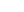, then the dilation of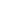by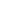defined by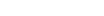is also Lebesgue measurable and has measure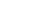14. More generally, if T is a linear transformation
Linear transformation
In mathematics, a linear map, linear mapping, linear transformation, or linear operator is a function between two vector spaces that preserves the operations of vector addition and scalar multiplication. As a result, it always maps straight lines to straight lines or 0...

and A is a measurable subset of Rn, then T(A) is also Lebesgue measurable and has the measure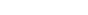.

All the above may be succinctly summarized as follows:
The Lebesgue measurable sets form a σ-algebra
Sigma-algebra
In mathematics, a σ-algebra is a technical concept for a collection of sets satisfying certain properties. The main use of σ-algebras is in the definition of measures; specifically, the collection of sets over which a measure is defined is a σ-algebra...

Complete measure
In mathematics, a complete measure is a measure space in which every subset of every null set is measurable...

translation-invariant measure
Measure (mathematics)
In mathematical analysis, a measure on a set is a systematic way to assign to each suitable subset a number, intuitively interpreted as the size of the subset. In this sense, a measure is a generalization of the concepts of length, area, and volume...

on that σ-algebra with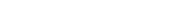The Lebesgue measure also has the property of being σ-finite
Sigma-finite measure
In mathematics, a positive measure μ defined on a σ-algebra Σ of subsets of a set X is called finite if μ is a finite real number . The measure μ is called σ-finite if X is the countable union of measurable sets of finite measure...

.

## Null sets

A subset of Rn is a null set if, for every ε > 0, it can be covered with countably many products of n intervals whose total volume is at most ε. All countable sets are null sets.

If a subset of Rn has Hausdorff dimension
Hausdorff dimension
thumb|450px|Estimating the Hausdorff dimension of the coast of Great BritainIn mathematics, the Hausdorff dimension is an extended non-negative real number associated with any metric space. The Hausdorff dimension generalizes the notion of the dimension of a real vector space...

less than n then it is a null set with respect to n-dimensional Lebesgue measure. Here Hausdorff dimension is relative to the Euclidean metric on Rn (or any metric Lipschitz
Lipschitz
- People :Lipschitz is a surname, which may be derived from the Polish city of Głubczyce , and may refer to:* Daniel Lipšic, Minister of Interior in Slovakia* Dr...

equivalent to it). On the other hand a set may have topological dimension less than n and have positive n-dimensional Lebesgue measure. An example of this is the Smith–Volterra–Cantor set which has topological dimension 0 yet has positive 1-dimensional Lebesgue measure.

In order to show that a given set A is Lebesgue measurable, one usually tries to find a "nicer" set B which differs from A only by a null set (in the sense that the symmetric difference
Symmetric difference
In mathematics, the symmetric difference of two sets is the set of elements which are in either of the sets and not in their intersection. The symmetric difference of the sets A and B is commonly denoted by A\,\Delta\,B\,orA \ominus B....

(AB)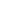(BA) is a null set) and then show that B can be generated using countable unions and intersections from open or closed sets.

## Construction of the Lebesgue measure

The modern construction of the Lebesgue measure is an application of Carathéodory's extension theorem
Carathéodory's extension theorem
In measure theory, Carathéodory's extension theorem states that any σ-finite measure defined on a given ring R of subsets of a given set Ω can be uniquely extended to the σ-algebra generated by R...

. It proceeds as follows.

Fix . A box in Rn is a set of the form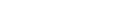where . The volume vol(B) of this box is defined to be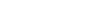For any subset A of Rn, we can define its outer measure
Outer measure
In mathematics, in particular in measure theory, an outer measure or exterior measure is a function defined on all subsets of a given set with values in the extended real numbers satisfying some additional technical conditions. A general theory of outer measures was first introduced by...

λ*(A) by: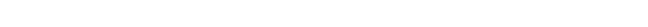We then define the set A to be Lebesgue measurable if for every subset S of Rn,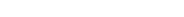These Lebesgue measurable sets form a σ-algebra, and the Lebesgue measure is defined by for any Lebesgue measurable set A.

The existence of sets that are not Lebesgue measurable is a consequence of a certain set-theoretical axiom
Axiom
In traditional logic, an axiom or postulate is a proposition that is not proven or demonstrated but considered either to be self-evident or to define and delimit the realm of analysis. In other words, an axiom is a logical statement that is assumed to be true...

, the axiom of choice, which is independent from many of the conventional systems of axioms for set theory
Set theory
Set theory is the branch of mathematics that studies sets, which are collections of objects. Although any type of object can be collected into a set, set theory is applied most often to objects that are relevant to mathematics...

. The Vitali theorem
Vitali set
In mathematics, a Vitali set is an elementary example of a set of real numbers that is not Lebesgue measurable, found by . The Vitali theorem is the existence theorem that there are such sets. There are uncountably many Vitali sets, and their existence is proven on the assumption of the axiom of...

, which follows from the axiom, states that there exist subsets of R that are not Lebesgue measurable. Assuming the axiom of choice, non-measurable set
Non-measurable set
In mathematics, a non-measurable set is a set whose structure is so complicated that it cannot be assigned any meaningful measure. Such sets are constructed to shed light on the notions of length, area and volume in formal set theory....

s with many surprising properties have been demonstrated, such as those of the Banach–Tarski paradox
The Banach–Tarski paradox is a theorem in set theoretic geometry which states the following: Given a solid ball in 3-dimensional space, there exists a decomposition of the ball into a finite number of non-overlapping pieces , which can then be put back together in a different way to yield two...

.

In 1970, Robert M. Solovay
Robert M. Solovay
Robert Martin Solovay is an American mathematician specializing in set theory.Solovay earned his Ph.D. from the University of Chicago in 1964 under the direction of Saunders Mac Lane, with a dissertation on A Functorial Form of the Differentiable Riemann–Roch theorem...

showed that the existence of sets that are not Lebesgue measurable is not provable within the framework of Zermelo–Fraenkel set theory
Zermelo–Fraenkel set theory
In mathematics, Zermelo–Fraenkel set theory with the axiom of choice, named after mathematicians Ernst Zermelo and Abraham Fraenkel and commonly abbreviated ZFC, is one of several axiomatic systems that were proposed in the early twentieth century to formulate a theory of sets without the paradoxes...

in the absence of the axiom of choice (see Solovay's model).

## Relation to other measures

The Borel measure agrees with the Lebesgue measure on those sets for which it is defined; however, there are many more Lebesgue-measurable sets than there are Borel measurable sets. The Borel measure is translation-invariant, but not complete
Complete measure
In mathematics, a complete measure is a measure space in which every subset of every null set is measurable...

.

The Haar measure
Haar measure
In mathematical analysis, the Haar measure is a way to assign an "invariant volume" to subsets of locally compact topological groups and subsequently define an integral for functions on those groups....

can be defined on any locally compact group
Topological group
In mathematics, a topological group is a group G together with a topology on G such that the group's binary operation and the group's inverse function are continuous functions with respect to the topology. A topological group is a mathematical object with both an algebraic structure and a...

and is a generalization of the Lebesgue measure (Rn with addition is a locally compact group).

The Hausdorff measure
Hausdorff measure
In mathematics a Hausdorff measure is a type of outer measure, named for Felix Hausdorff, that assigns a number in [0,∞] to each set in Rn or, more generally, in any metric space. The zero dimensional Hausdorff measure is the number of points in the set or ∞ if the set is infinite...

is a generalization of the Lebesgue measure that is useful for measuring the subsets of Rn of lower dimensions than n, like submanifold
Submanifold
In mathematics, a submanifold of a manifold M is a subset S which itself has the structure of a manifold, and for which the inclusion map S → M satisfies certain properties. There are different types of submanifolds depending on exactly which properties are required...

s, for example, surfaces or curves in R³ and fractal
Fractal
A fractal has been defined as "a rough or fragmented geometric shape that can be split into parts, each of which is a reduced-size copy of the whole," a property called self-similarity...

sets. The Hausdorff measure is not to be confused with the notion of Hausdorff dimension
Hausdorff dimension
thumb|450px|Estimating the Hausdorff dimension of the coast of Great BritainIn mathematics, the Hausdorff dimension is an extended non-negative real number associated with any metric space. The Hausdorff dimension generalizes the notion of the dimension of a real vector space...

.

It can be shown that there is no infinite-dimensional analogue of Lebesgue measure
There is no infinite-dimensional Lebesgue measure
In mathematics, it is a theorem that there is no analogue of Lebesgue measure on an infinite-dimensional Banach space. Other kinds of measures are therefore used on infinite-dimensional spaces: often, the abstract Wiener space construction is used...

.